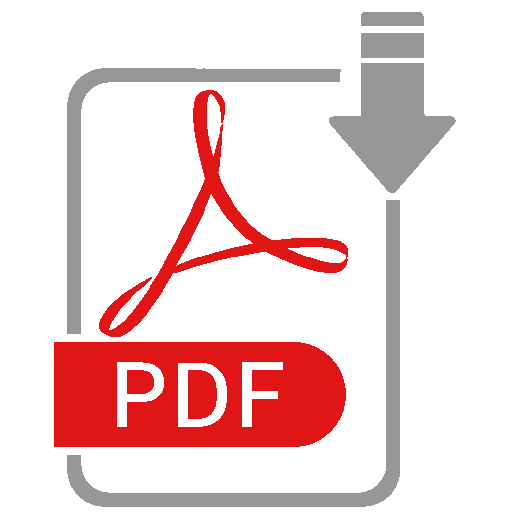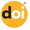Previous issues

Development and evaluation of equation to predict live weight in crossbred heifers using hip widthDownload paperVer Versión Españolahttps://doi.org/10.12706/itea.2018.022

 Authors: S. Herrera‑López, R. García‑Herrera, A.J. Chay‑Canul, M. González‑Ronquillo, U. Macías‑Cruz, V.F. Díaz‑Echeverría, F. Casanova‑Lugo y A. Piñeiro‑Vázquez Issue: 114-4 (368-377) Topic: Animal Production Keywords: body weight, body measurements, tropical heifers, mathematical models Summary: The aim of this study was to evaluate the relationship between body weight (BW), and the hip width (HW) in crossbred heifers (n = 500), reared under conditions of humid tropics in Mexico. The relationship between the body weight and hip width was estimated using nonlinear regression models. The correlation coefficient between the body weight and hip width was 0.98 (p < 0.001). The body weight ranged from 105.00 to 695 kg, while the hip width ranged from 23.00 to 65.50 cm. The correlation coefficient between the body weight and the hip width was 0.98 (p < 0.001). The equation was BW (kg) = 0.2615 (±0.0167)* HW1.902(±0.0164) (P < 0.0001, r² = 0.97, Mean Square Error = 554.52, Residual Standard Deviation = 23.55 and n = 500). The evaluation of the equation was assessed with the Model Evaluation System using data obtained from 70 heifers of a similar condition. Linear regression analysis between body weight values observed and predicted showed a high correlation (r = 0.98) and that the intercept and slope were not different to 0 and 1 respectively (P > 0.05), as well as the equation explains 97% of the variance (r² = 0.97). The parameters of precision and accuracy showed that the equation proposal presented a high precision (r² = 0.97), accuracy (Cb = 0.99) and reproducibility (CCC = 0.97). The equation had a high efficiency prediction (MEF = 0.96). The root square of the mean square of error of prediction (RMSEP) accounted for 8.11% of the body weight observed. The prediction equation evaluated showed good precision and accuracy to predict the body weight of crossbred heifers. The measurement of hip width can represent an easier option to get to estimate the body weight in this type of animal.
Back to Issue 114-4

DATABASES AND REPOSITORIES